# 国立情報学研究所 - ディジタル・シルクロード・プロジェクト 『東洋文庫所蔵』貴重書デジタルアーカイブ

 ディジタル・シルクロード > 東洋文庫アーカイブ > VIII-5-B2-11 > V-1 > カラー画像
 カラー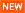IIIFカラー高解像度 白黒高解像度 PDF 日本語 English
0135 Memoir on Maps of Chinese Turkistan and Kansu : vol.1 中国領トルキスタンおよび甘粛の地図に関する覚書 : vol.1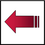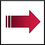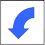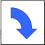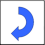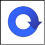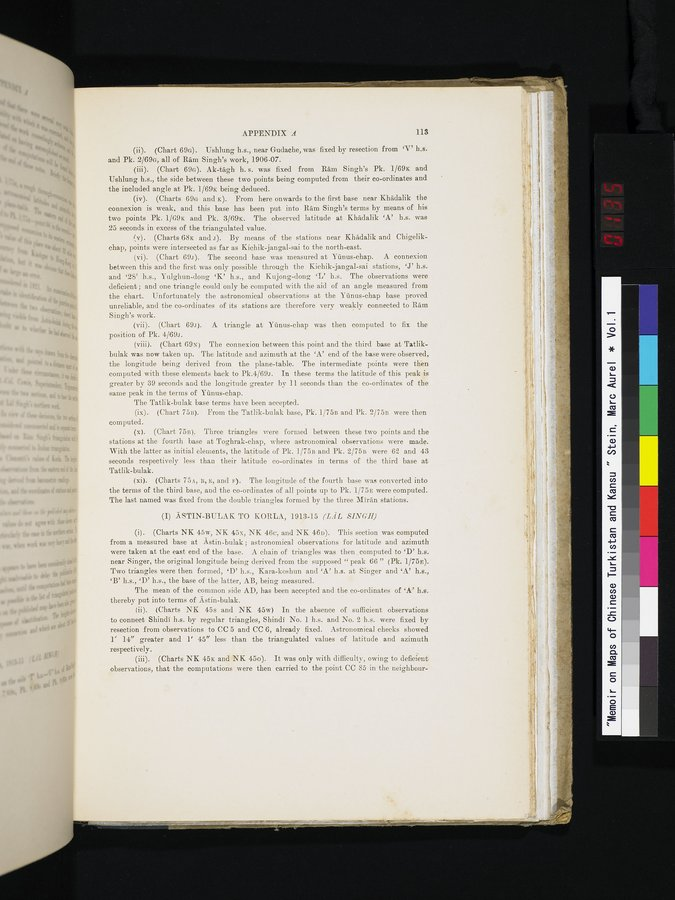### OCR読み取り結果

APPENDIX A   113

1.  (Chart 69G). Ushlung h.s., near Gudache, was fixed by resection from `V' h.s. and Pk. 2/69G, all of Ram Singh's work, 1906-07.

2.  (Chart 69G). Ak-tàgh h. s. was fixed from Ram Singh's Pk. 1/69x and Ushlung h.s., the side between these two points being computed from their co-ordinates and the included angle at Pk. 1/69x being deduced.

3.  (Charts 69G and x). From here onwards to the first base near Khàdalik the connexion is weak, and this base has been put into Ram Singh's terms by means of his two points Pk. 1/69E. and Pk. 3/69x. The observed latitude at Khàdalik `A' h.s. was 25 seconds in excess of the triangulated value.

4.  (Charts 68x and r). By means of the stations near Khàdalik and Chigelikchap, points were intersected as far as Kichik-jangal-sai to the north-east.

5.  (Chart 69r). The second base was measured at Yunus-chap.. A connexion between this and the first was only possible through the Kichik-jangal-sai stations, 'J' h.s. and `28' h.s., Yulghun-dong 'K' h.s., and Kujong-dong 'L' h.s. The observations were deficient ; and one triangle could only be computed with the aid of an angle measured from the chart. Unfortunately the astronomical observations at the Yunus-chap base proved unreliable, and the co-ordinates of its stations are therefore very weakly connected to Ram Singh's work.

6.  (Chart 694 A triangle at Yantis-chap was then computed to fix the position of Pk. 4/69r.

7. (Chart 69N) The connexion between this point and the third base at Tatlikbulak was now taken up. The latitude and azimuth at the `A' end of the base were observed, the longitude being derived from the plane-table. The intermediate points were then computed with these elements back to Pk.4/69r. In these terms the latitude of this peak is greater by 39 seconds and the longitude greater by 11 seconds than the co-ordinates of the same peak in the terms of Yunus-chap.

The Tatlik-bulak base terms have been accepted.

1.  (Chart 75B). From the Tatlik-bulak base, Pk. 1/75B and Pk. 2/75B were then computed.

2.  (Chart 75B). Three triangles were formed between these two points and the stations at the fourth base at Toghrak-chap, where astronomical observations were made. With the latter as initial elements, the latitude of Pk. 1/75B and Pk. 2/75B were 62 and 43 seconds respectively less than their latitude co-ordinates in terms of the third base at Tatlik-bulak.

3.  (Charts 75A, B, E, and F). The longitude of the fourth base was converted into the terms of the third base, and the co-ordinates of all points up to Pk. 1/75E were computed. The last named was fixed from the double triangles formed by the three Miràn stations.

(I) ASTIN-BULAK TO KORLA, 1913-15 (LA L SINGH)

1.  (Charts NK 45w, NK 45x, NK 46c, and NK 46D). This section was computed from a measured base at Astin-bulak; astronomical observations for latitude and azimuth were taken at the east end of the base. A chain of triangles was then _computed to `D' h.s. near Singer, the original longitude being derived from the supposed " peak 66 " (Pk. 1/75E). Two triangles were then formed, 'D' h.s., Kara-koshun and 'A' h.s. at Singer and 'A' h.s., `B' h.s., `D' h.s., the base of the latter, AB, being measured.

The mean of the common side AD, has been accepted and the co-ordinates of 'A' h.s. thereby put into terms of Astin-bulak.

1.  (Charts NE 45s and NK 45w) In the absence of sufficient observations to connect Shindi h.s. by regular triangles, Shindi No. 1 h.s. and No. 2'h.s. were fixed by resection from observations to CC 5 and CC .6, already fixed. Astronomical checks showed 1' 14" greater and 1' 45" less than the triangulated values of latitude and azimuth respectively.

2.  (Charts NK 45x and NK 45o). It was only with difficulty, owing to deficient observations, that the computations were then carried to the point CC 85 in the neighbour-